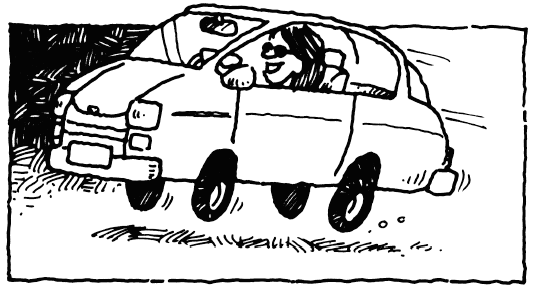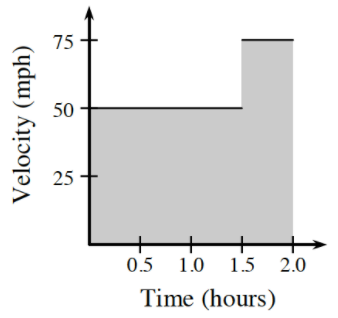### Home > PC3 > Chapter 1 > Lesson 1.3.2 > Problem1-128

1-128.Allison drove for a total of $2$ hours. She drove $50$ mph for the first $90$ minutes and then drove $75$ mph for the last $30$ minutes.

1. How far did she travel?

What is distance in terms of rate and time? Be careful with the units. Convert minutes to hours since the rate is in miles per hour.
The distance Allison drove in the first $90$ minutes is shown below. Finish the calculations.
$50$ mph ($1.5$ h) + ...

2. Sketch a graph of this information using velocity in miles per hour and time in hours. Shade the area in the first quadrant that is under the graph. Describe the shape(s) of the shaded region.3. What is the area of the shaded region?

There are two rectangles? What is the area of each rectangle?

4. What units are used for the area? Use the units for the height and base of the rectangles to justify your answer.

What are the units of the $x$-axis and the $y$-axis?

$\frac{\text{miles}}{\text{hour}} \cdot \text{hours}$